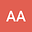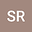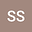Solutions of sum-type singular fractional q-integro-differential equation with $m$-point boundary value using quantum calculus
•••• +1
Mediterranean University of Reggio Calabria Department of Informatics Mathematics Electronics and Transport

Author ProfileSoheil Salahshour
Bahcesehir Universitesi
Author ProfileBu Ali Sina University
Author Profile## Abstract

In this study, we investigate the sum-type singular nonlinear fractional q-integro-differential $m$-point boundary value problem. The existence of positive solutions is obtained by the properties of the Green function, standard Caputo $q$-derivative, Riemann-Liouville fractional $q$-integral and the means of a fixed point theorem on a real Banach space $(\mathcal{X}, \|.\|)$ which has a partially order by using a cone $P \subset \mathcal{X}$. The proofs are based on solving the operator equation $\mathcal{O}_1 x + \mathcal{O}_2 x = x$ such that the operator $\mathcal{O}_1$, $\mathcal{O}_2$ are $r$-convex, sub-homogeneous, respectively and define on cone $P$. As applications, we provide an example illustrating the primary effects.
20 Jan 2020Submitted to Mathematical Methods in the Applied Sciences
25 Jan 2020Submission Checks Completed
25 Jan 2020Assigned to Editor
25 Jan 2020Reviewer(s) Assigned
01 Feb 2020Review(s) Completed, Editorial Evaluation Pending
02 Feb 2020Editorial Decision: Revise Major